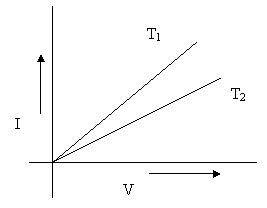Courses

# Test: Ohm’S Law

## 5 Questions MCQ Test Physics Class 12 | Test: Ohm’S Law

Description
This mock test of Test: Ohm’S Law for Class 12 helps you for every Class 12 entrance exam. This contains 5 Multiple Choice Questions for Class 12 Test: Ohm’S Law (mcq) to study with solutions a complete question bank. The solved questions answers in this Test: Ohm’S Law quiz give you a good mix of easy questions and tough questions. Class 12 students definitely take this Test: Ohm’S Law exercise for a better result in the exam. You can find other Test: Ohm’S Law extra questions, long questions & short questions for Class 12 on EduRev as well by searching above.
QUESTION: 1

### V-I graph of which material shows the straight line

Solution:

Materials which obeys the ohm’s law show straight line in the V-I graph. Since silver is the only ohmic material in given options, it shows straight line curve.

QUESTION: 2

### The following fig. shows I-V graph for a given metallic wire at two temperatures T1and T2.Then,​

Solution:

The correct option is Option B.

The slope of the graph = I / V

In case of T2, I/V is less. Hence, V/I (=R) is more.

Value of resistance increases with increasing temperature.

Hence, T2 >T

QUESTION: 3

### How many electrons are flowing per second through a section of a conductor corresponding to current of 1A?​

Solution:

I=Q/t
1 Ampere = 1 Coulomb /1 Secon
Charge on 1 electron = 1.6 x 10^-19
Coulomb
By unitary method,
If 1.6 x 10^-19 Coulomb / Second (Ampere) = Current by 1 electron
then, 1( Coulomb / Second) or (Ampere) = 1 / (1.6 x 10^-19) electrons
i.e, 6.25 x 10^18 electrons.

QUESTION: 4

1 ohm is equal to

Solution:

1 Ohm is defined as the resistance of a conductor with a potential difference of 1 volt applied to the ends through which 1-ampere current flows.
Ohms is the SI unit of electrical resistance.

QUESTION: 5

Which of the following is an ohmic conductor?

Solution:

Ohmic conductors are those which follows ohm's Law

Constantan is an copper nickle alloy which follows ohm's law

An electrolyte is a chemical that produces an electrically conducting solution and hence conducts electrical current.

But Electrolyte can be  Ohmic as well as non-ohmic conductor

Transistor is semi-conductor device and does not follow ohm's Law

thermionic valves  - is vacuum tube (electronic tube) , uses ion emission.

So option C Constanton s an ohmic conductor.